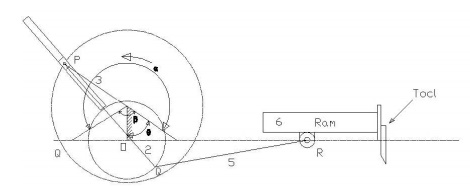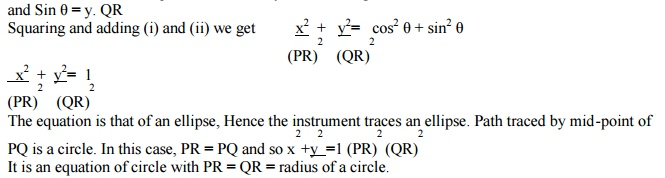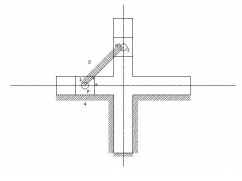Home | | Kinematics of Machinery | Whitworth quick return motion mechanism

# Whitworth quick return motion mechanism

Third inversion is obtained by fixing the crank i.e. link 2. Whitworth quick return mechanism is an application of third inversion. This mechanism is shown in the figure below. The crank OC is fixed and OQ rotates about O.

Whitworth quick return motion mechanism:Third inversion is obtained by fixing the crank i.e. link 2. Whitworth quick return mechanism is an application of third inversion. This mechanism is shown in the figure below. The crank OC is fixed and OQ rotates about O. The slider slides in the slotted link and generates a circle of radius CP. Link 5 connects the extension OQ provided on the opposite side of the link 1 to the ram (link 6). The rotary motion of P is taken to the ram R which reciprocates. The quick return motion mechanism is used in shapers and slotting machines. The angle covered during cutting stroke from P1 to P2 in counter clockwise direction is α or 360 -2θ. During the return stroke, the angle covered is 2θ or β.

Therefore,1. Rotary engine mechanism or Gnome Engine:

Rotary engine mechanism or gnome engine is another application of third inversion. It is a rotary cylinder V – type internal combustion engine used as an aero – engine. But now Gnome engine has been replaced by Gas turbines. The Gnome engine has generally seven cylinders in one plane. The crank OA is fixed and all the connecting rods from the pistons are connected to A. In this mechanism when the pistons reciprocate in the cylinders, the whole assembly of cylinders, pistons and connecting rods rotate about the axis O, where the entire mechanical power developed, is obtained in the form of rotation of the crank shaft. This mechanism is shown in the figure below.2   Double Slider Crank Chain:

A four bar chain having two turning and two sliding pairs such that two pairs of the same kind are adjacent is known as double slider crank chain.

3   Inversions of Double slider Crank chain:

It consists of two sliding pairs and two turning pairs. They are three important inversions of double slider crank chain. 1) Elliptical trammel. 2) Scotch yoke mechanism. 3) Oldham’s Coupling.

4. Elliptical Trammel:

This is an instrument for drawing ellipses. Here the slotted link is fixed. The sliding block P and Q in vertical and horizontal slots respectively. The end R generates an ellipse with the displacement of sliders P and Q.The co-ordinates of the point R are x and y. From the fig. cos θ = x.PR

The equation is that of an ellipse, Hence the instrument traces an ellipse. Path traced by mid-point of PQ is a circle. In this case, PR = PQ and soIt is an equation of circle with PR = QR = radius of a circle.5. Scotch yoke mechanism: This mechanism, the slider P is fixed. When PQ rotates above P, the slider Q reciprocates in the vertical slot. The mechanism is used to convert rotary to reciprocating mechanism.5.Oldham’s coupling: The third inversion of obtained by fixing the link connecting the 2 blocks P & Q. If one block is turning through an angle, the frame and the other block will also turn through the same angle. It is shown in the figure below.An application of the third inversion of the double slider crank mechanism is Oldham’s coupling shown in the figure. This coupling is used for connecting two parallel shafts when the distance between the shafts is small. The two shafts to be connected have flanges at their ends, secured by forging. Slots are cut in the flanges. These flanges form 1 and 3. An intermediate disc having tongues at right angles and opposite sides is fitted in between the flanges. The intermediate piece forms the link 4 which slides or reciprocates in flanges 1 & 3. The link two is fixed as shown. When flange 1 turns, the intermediate disc 4 must turn through the same angle and whatever angle 4 turns, the flange 3 must turn through the same angle. Hence 1, 4 & 3 must have the same angular velocity at every instant. If the distance between the axis of the shaft is x, it will be the diameter if the circle traced by the centre of the intermediate piece. The maximum sliding speed of each tongue along its slot is given by

v=xω where, ω = angular velocity of each shaft in rad/sec v = linear velocity in m/sec